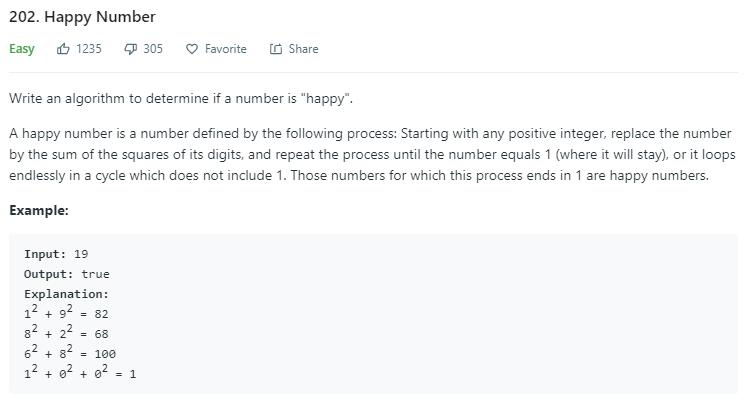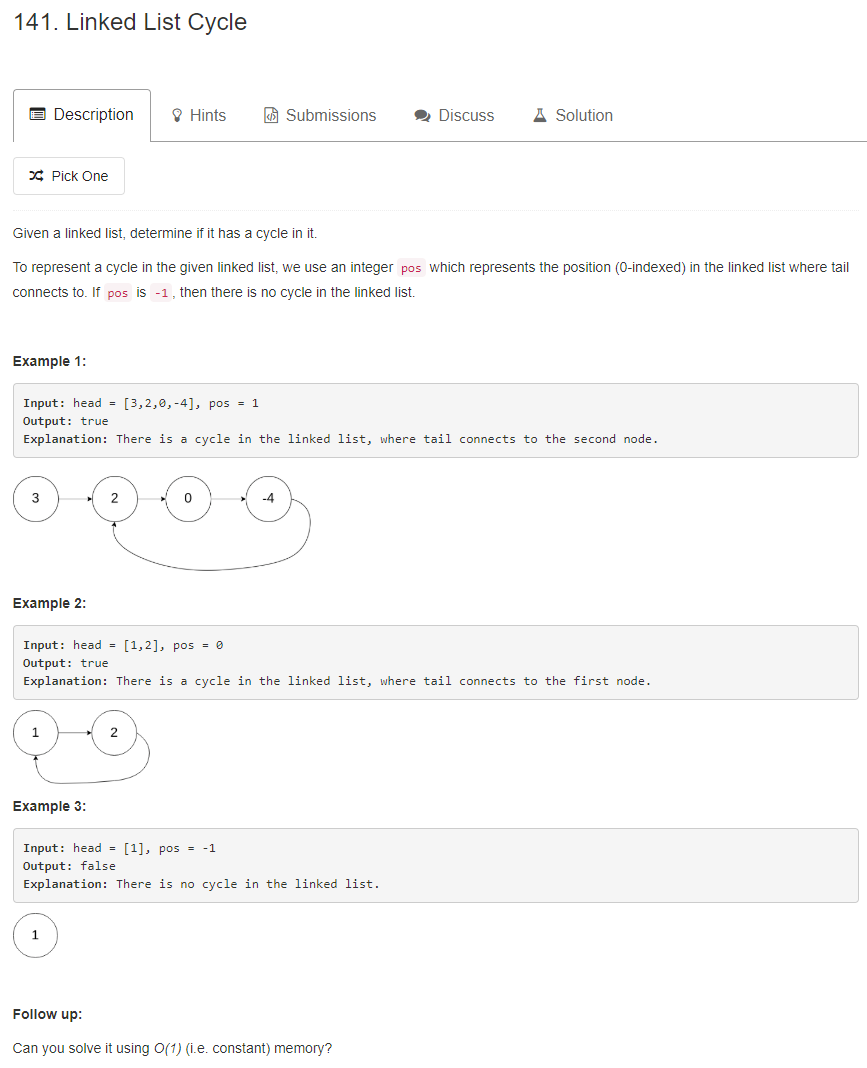# 题目描述（简单难度）# 解法一

public boolean isHappy(int n) {
HashSet<Integer> set = new HashSet<>();
while (true) {
int next = getNext(n);
if (next == 1) {
return true;
}
if (set.contains(next)) {
return false;
} else {
n = next;
}
}
}

//计算各个位的平方和
private int getNext(int n) {
int next = 0;
while (n > 0) {
int t = n % 10;
next += t * t;
n /= 10;
}
return next;
}


# 解法二public boolean isHappy(int n) {
int slow = n;
int fast = n;
do {
slow = getNext(slow);
fast = getNext(getNext(fast));
} while (slow != fast);
return slow == 1;
}

private int getNext(int n) {
int next = 0;
while (n > 0) {
int t = n % 10;
next += t * t;
n /= 10;
}
return next;
}